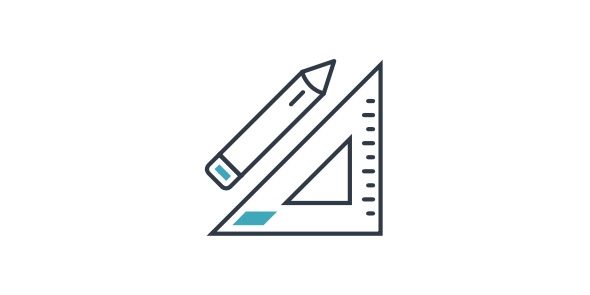# Math Quiz For 3rd Grade

14 Questions | Total Attempts: 117SettingsHow much do you know about 3rd grade math? Take this quiz and score a perfect!

• 1.
Which number is odd?
• A.

3

• B.

6

• C.

12

• D.

4

• 2.
What is the top number of a fraction called?
• A.

Denominator

• B.

Improper

• C.

Mixed Number

• D.

Numerator

• 3.
Which is larger: 4/7 or 6/7?
• A.

7-Jun

• B.

They Are Equal

• C.

6-Jul

• D.

7-Apr

• 4.
What is 300 minus 156?
• A.

145

• B.

144

• C.

146

• D.

143

• 5.
If you buy a pencil that costs \$.26 and pay for it with a dollar bill, how much change will you get?
• A.

76 Cents

• B.

74 Cents

• C.

86 Cents

• D.

84 Cents

• 6.
Which number is even?
• A.

4

• B.

1

• C.

9

• D.

3

• 7.
What is the bottom number of a fraction called?
• A.

Improper

• B.

Mixed Number

• C.

Denominator

• D.

Numerator

• 8.
• A.

An Even Number

• B.

A Smaller Number

• C.

A Fractional Number

• D.

An Odd Number

• 9.
What number must be added to 16 in order to get 31?
• A.

15

• B.

25

• C.

26

• D.

16

• 10.
What place is the 2 in for the number 120,000?
• A.

Tens

• B.

Thousands

• C.

Hundreds

• D.

Ten Thousands

• 11.
What is 49 divided by 7?
• A.

343

• B.

42

• C.

56

• D.

7

• 12.
How many days are there in a leap year?
• A.

52

• B.

365

• C.

364

• D.

366

• 13.
What is the product of 12 multiplied by 6?
• A.

126

• B.

18

• C.

72

• D.

621

• 14.
What number must be added to 131 to get 271?
• A.

140

• B.

130

• C.

160

• D.

150

Related Topics